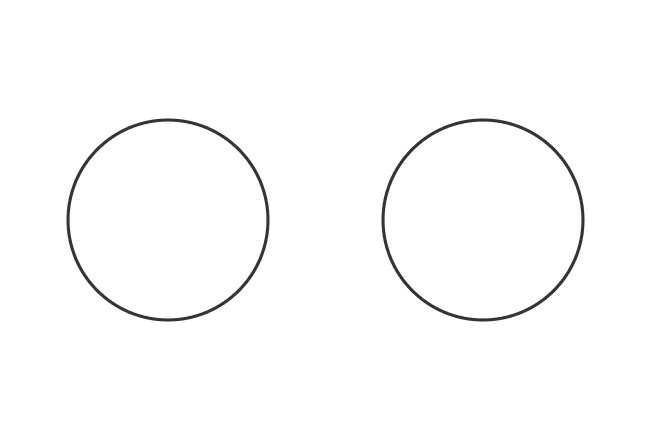# Mixed fractions

A mixer of a whole quantity and a fraction is called a mixed fraction.

## Introduction

A mixed fraction is basically a fraction but it consists of two parts, which are an integer part and a proper fraction part. You may wonder by seeing the numbers in the following special form.

$1\dfrac{3}{4}, 2\dfrac{1}{6}, -5\dfrac{5}{8}, \ldots$

The all of them are representing mixed fractions numerically.

In mathematics, the mixed fractions are formed by the mixer of a proper fraction with one or more whole quantities. If a proper fraction is added to a whole quantity, then the quantity becomes a fraction. Hence, the fractions are called as the mixed fractions.

Remember, the quantity of a mixed fraction is always greater than one. So, they are basically improper fractions but all improper fractions are not mixed fractions.

### Examples

Let us understand the concept of mixed fractions from the following examples.

#### Example – 1

Consider two circles and divide a circle into three equal parts. Now, take a circle and two parts from the three parts of another circle as a fraction.Due to mixing a fraction with a whole quantity, a mixed fraction is formed and it can be calculated mathematically.

1. The quantity of a full circle is $1$.
2. The quantity of the fraction of another circle is $\large \frac{2}{3}$.

The sum of the quantities is equal to the mixed fraction.

$\implies$ Mixed fraction $\,=\,$ $1+\dfrac{2}{3}$

It is simply expressed in the following special form in mathematics.

$\,\,\, \therefore \,\,\,\,\,\,$ Mixed fraction $\,=\,$ $1\dfrac{2}{3}$

#### Example – 2

Take four rectangles but split a rectangle as five equal parts. Now, take three rectangles and three parts from five parts of another rectangle as a fraction.Their mixer forms a mixed fraction, which can be evaluated mathematically.

1. The quantity of whole rectangles is $3$.
2. The quantity of the fraction of remaining rectangle is $\large \frac{3}{5}$.

Let’s calculate the mixed fraction.

$\implies$ Mixed fraction $\,=\,$ $3+\dfrac{3}{5}$

$\,\,\, \therefore \,\,\,\,\,\,$ Mixed fraction $\,=\,$ $3\dfrac{3}{5}$

Thus, the mixed fractions are formed geometrically and also above steps are used to calculate them mathematically.

Latest Math Topics
Jun 26, 2023
Jun 23, 2023

###### Math Questions

The math problems with solutions to learn how to solve a problem.

Learn solutions

Practice now

###### Math Videos

The math videos tutorials with visual graphics to learn every concept.

Watch now

###### Subscribe us

Get the latest math updates from the Math Doubts by subscribing us.